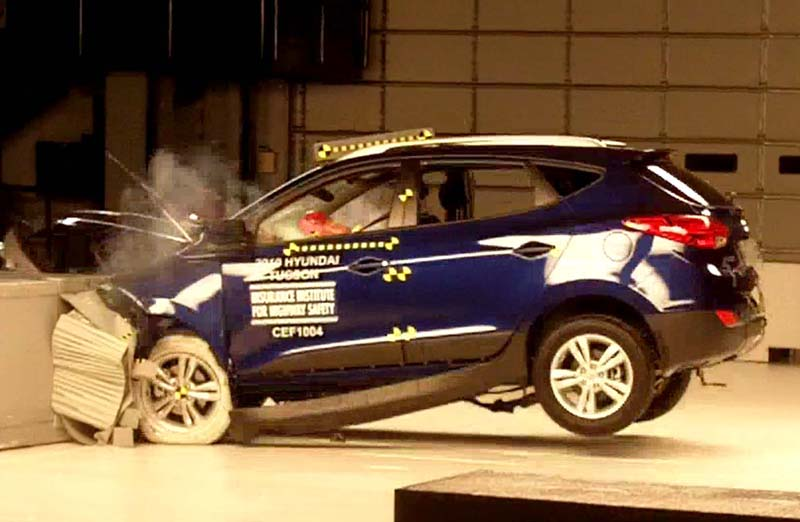# It's gonna crash!In a crash test, a $1.5$ metric ton vehicle collides a brick wall. The initial velocity of the vehicle is $v_{i}=-10\text{ m/s}$ while its final velocity is $v_{f}=2.6\text{ m/s}$. If the collision lasts for $0.2$ seconds, what is the average force (in Newtons) exerted on the vehicle due to the collision?

×